First-order first-degree autonomous differential equation

(diff) ← Older revision | Latest revision (diff) | Newer revision → (diff)

Definition

Following the convention for autonomous differential equation, we denote the dependent variable by$x$ and independent variable by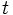$t$.

Form of the differential equation

The differential equation is of the form:$\frac{dx}{dt} = f(x)$

Solution method and formula

We convert the differential equation to an integration problem:$\int \frac{dx}{f(x)} = \int dt$

and carry out the integrations on both sides. If$p$ is an antiderivative of$1/f$, the solution will be:$p(x) = t + C$

with$C$ the freely varying parameter over$\R$: every particular value of$C$ gives a solution. To express$x$ as a function of$t$, we need to invert$p$. If we can do so, we'd have: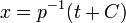$x = p^{-1}(t + C)$

as the general solution.

In addition, there may be stationary solutions. These are solutions that correspond to constant functions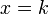$x = k$ that satisfy$f(k) = 0$.

Analysis

Starting at time zero with value one

Suppose we want to solve the initial value problem for the differential equation:$\frac{dx}{dt} = f(x)$

subject to the initial condition that at$t = 0$,$x = 1$.

We consider various possibilities for$f$ a function that sends 1 and higher numbers to positive numbers, and make cases based on the growth rate of$f$:

Nature of$f$ Nature of$x$ in terms of$t$?
constant function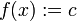$f(x) := c$ linear function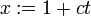$x := 1 + ct$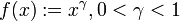$f(x) := x^\gamma, 0 < \gamma < 1$$x = O(t^{1/(1 - \gamma)})$ (i.e., it grows roughly like a power function of$t$)
linear function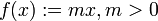$f(x) := mx, m > 0$ exponential function$x := \exp(mt)$
linear times logarithmic, something like$x (\ln x + 1)$$x$ grows something like a doubly exponential function of$t$ (note: if we wanted growth between exponential and double exponential, we would need something like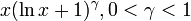$x(\ln x + 1)^\gamma, 0 < \gamma < 1$, and if we wanted triple exponential growth, we would multiply by a double logarithmic term)$f(x) := x^\gamma, \gamma > 1$$x$ grows so fast in terms of$t$ that it reaches$\infty$ in finite time.